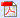## Aug 31, 2009

1. Write the expanded form of 3456.

a. 6000+500+40+3

b. 3+40+500+6000

c. 3000+400+50+6

d. 3+4+5+6

2. Five-sevenths of the pens are green. Represent this as a fraction.

a. 5/7

b. 5.7

c. 5-7

d. 77777

3. 1 quarter=___ cents

a. 5

b. 10

c. 20

d. 25

4. There are 28 candies. If each kid gets 4 candies, find the number of kids.

a. 5

b. 6

c. 7

d. 8

5. Which of the following statement is false?

a. 12 gallons of milk

b. 7 grams of temperature

c. 5 miles of away

d. 3 kg of sugar

6. Identify the time in the given clock.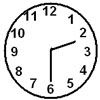a. 2:06

b. 6:02

c. 2:30

d. 6:10

7. Which of the following is better estimate to measure the length of your computer table?

a. 1.5 miles

b. 1.5 meter

c. 1.5 kilometer

d. 1.5 inches

8. Which of the following is more?

a. 12 grams

b. 12 kilograms

c. 12 ounces

d. 12 pounds

9. A water tank is of length 6 m and width 4m. Find the perimeter of the water tank.

a. 10 m

b. 20 m

c. 24 m

d. 30 m

10. The angle made by a straight line is _____ degree

a. 90

b. 100

c. 120

d. 180

11. Which of the following alphabet satisfies line of symmetry?

a. R

b. M

c. J

d. F

12. Which of the following is a pentagon?

a.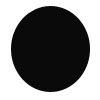b.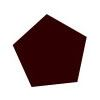c.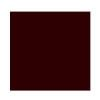d.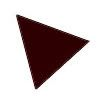13. Complete the pattern:

24, 20, 16, 12, ____, 4.

a. 13

b. 10

c. 8

d. 6

14. If ∆+5=8, then ∆ is ____

a. 1

b. 2

c. 3

d. 4

15. Y plus 4.

How do you write the above sentence as a mathematical expression?

a. y-4

b. y+4

c. y4

d. y and 4

16. 425+125=__ +100

Which of the following number makes the above equation true?

a. 550

b. 450

c. 450

d. 350

17. Find the mode of the following sequence:

3,6,2,4,3,4,5,6,8,6.

a. 3

b. 4

c. 6

d. 8

18. A survey was conducted among grade 3 students of St. Thomas School to find their favorite colors. From the given bar graph, find the number of students who likes green color?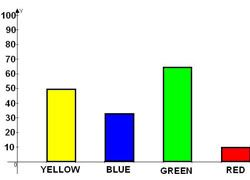a. 50

b. 60

c. 70

d. 80

19. In a box there are 3 red balls and 9 blue balls. The probability of choosing a blue ball is __________

a. More likely

b. Less likely

c. Equally likely

d. Unlikely

20. From the given table find the number of oranges.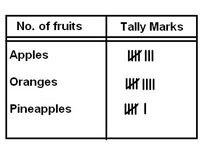a. 7

b. 8

c. 9

d. 10

Related articles:

Questions: